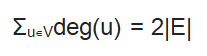# Handshaking Lemma in Graph Theory – Handshaking Theorem

Hello Everyone,

Today we will see Handshaking lemma associated with graph theory. Before starting lets see some terminologies.

Degree: It is a property of vertex than graph. Degree is a number of edges associated with a node.

Pendant vertices: Vertices with degree 1 are known as pendant vertices.

Isolated vertices: Vertices with degree 0 are known as Isolated vertices.

Now let us see the statement of the lemma first, It says:

In every finite undirected graph number of vertices with odd degree is always even.

Note: This theorem is only correct for undirected graphs with finite length.

The Handshaking lemma can be easily understood once we know about the degree sum formula. The degree sum formula says that:

The summation of degrees of all the vertices in an undirected graph is equal to twice the number of edges present in it.

It can be stated as:This is evident as every edge is associated with two nodes and will add 2 to the total summation.

Let’s take an example :In the above image the number of edges is 8, so |E| = 8.

Now,

deg(A) = 3

deg(B) = 2

deg(C) = 3

deg(D) = 2

deg(E) = 4

deg(F) = 2

Which sums upto 16 which is equal to 2*|E|.

Now let’s come to our original statement.

In every finite undirected graph number of vertices with odd degree is always even.

Now to understand this,

Lets write the above degree some formula as:Here k denotes vertices with odd degree and t denotes vertices with degree.

The summation of degrees of all the vertices with even degree will be even now remaining are the vertices with odd degree and as we know the total sum must be even so the summation of degrees of all the vertices having odd degree must be even. This is only possible if the number of vertices is even which proves our lemma.

The above lemma is very useful for proving some very interesting properties of trees and to understand different properties of cut vertices, Full and complete binary trees.